# Puzzler: Data exploration solution

This was a fun little puzzle. I wonder how many people got to the first image, but did not continue to the real solution!

### Load the data and take a first look

load puzzler.mat
plot(c,'.')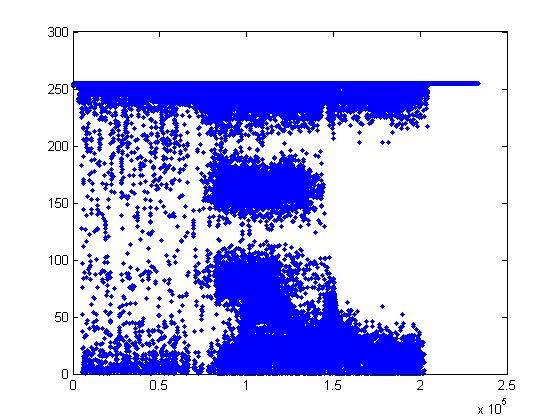### What is this stuff?

There is a lot of data, ranges from ~0 to ~255. Makes me think it is an image that has been made into a vector. How many different ways can this data be reshaped into a matrix?

dimensions = factor(numel(c))
dimensions =
419   557


### Reshape it!

What 'luck'! There is only one pair of prime factors. Makes you think this is the right path.

d = reshape(c, dimensions);
imagesc(d)
colormap(gray)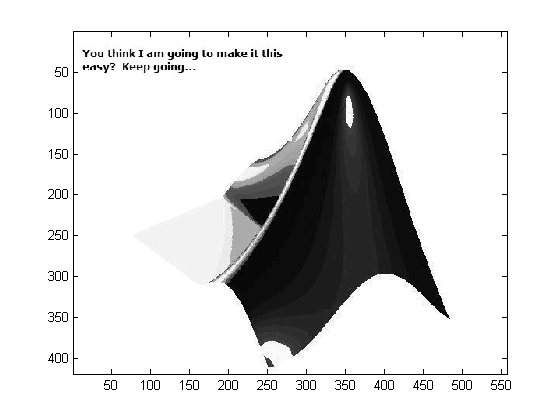### Tricky one.

We are on the right path, but not there yet. Those values were suspicious. Why were there decimal parts for an image. Let's look at the decimal parts.

e = rem(d,1);
imagesc(e)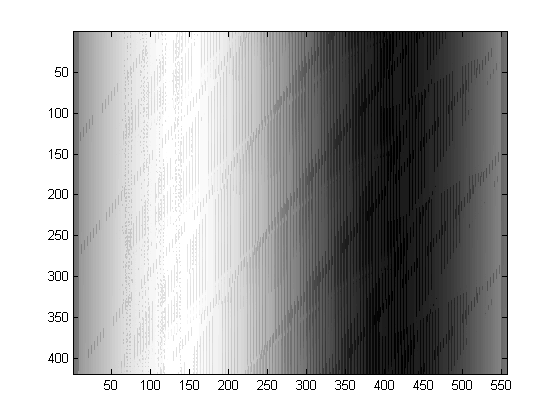### There is pattern here

This is the right path, there is pattern here

f = e(:);
plot(f,'.');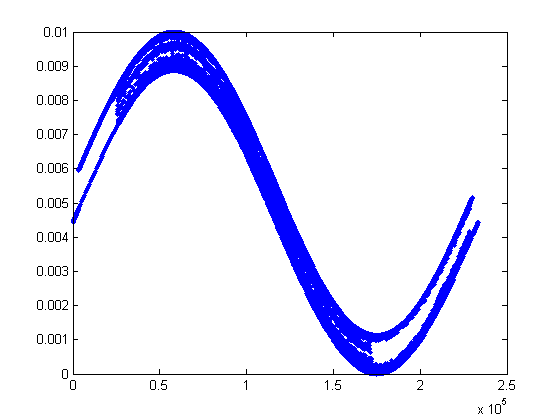### Lets try the other reshape

There are two possible reshapes, try the other

g = reshape(f, fliplr(dimensions));
imagesc(g)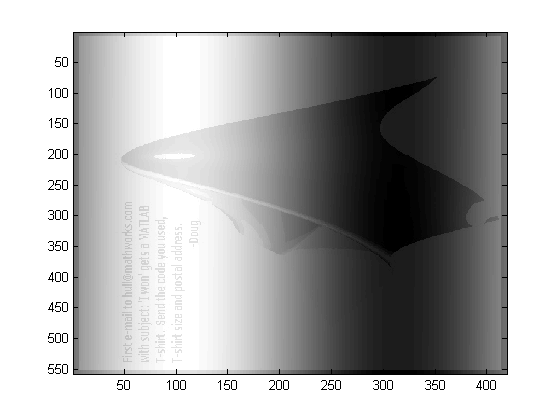### This is it

Now just clean it up a little

h = rot90(g, -1);
imagesc(h)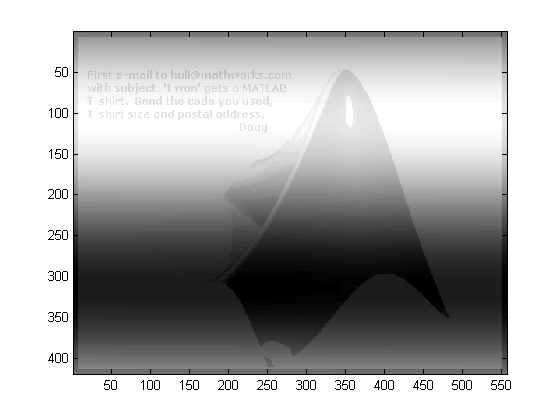Published with MATLAB® 7.6

|